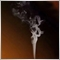# 为什么这个EA不能加仓？7

EA思路，在MA10、MA20、MA30上方，每收盘一根阳线，发一张多单，收盘价小于MA10时，多单平仓；在MA10、MA20、MA30下方，每收盘一根阴线，发一张空单，收盘价大于MA10时，空单平仓。但是目前只有在EA加载后，第一个信号发出时开仓，而不能加仓。求各位大神帮忙查看一下，感激不尽。

```//+------------------------------------------------------------------+
//|                                               Moving Average.mq4 |
//|                   Copyright 2005-2014, MetaQuotes Software Corp. |
//|                                              http://www.mql4.com |
//+------------------------------------------------------------------+
#property copyright   "2005-2014, MetaQuotes Software Corp."
#property description "Moving Average sample expert advisor"

#define MAGICMA  20131111
//--- Inputs
input double Lots          =0.02;
input double iLots         =0.02;  //追加订单开仓量
input int Order_Total      =1;     //最大持仓手数为1手
input double MaximumRisk   =0.02;
input double DecreaseFactor=3;
input int    MovingPeriod1  =10;
input int    MovingPeriod2  =20;
input int    MovingPeriod3  =30;
input int    MovingShift    =0;
input int    TicketNo;
input string ReturnMarketlnfomation;
input int    Open_New_Order;
//+------------------------------------------------------------------+
//| Calculate open positions                                         |
//+------------------------------------------------------------------+
int CalculateCurrentOrders(string symbol)
{
//---
for(int i=0;i<OrdersTotal();i++)
{
if(OrderSymbol()==Symbol() && OrderMagicNumber()==MAGICMA)
{
if(OrderType()==OP_SELL) sells++;
}
}
//--- return orders volume
else       return(-sells);
}
//+------------------------------------------------------------------+
//| Calculate optimal lot size                                       |
//+------------------------------------------------------------------+
double LotsOptimized()
{
double lot=Lots;
int    orders=HistoryTotal();     // history orders total
int    losses=0;                  // number of losses orders without a break
//--- select lot size
lot=NormalizeDouble(AccountFreeMargin()*MaximumRisk/1000.0,1);
//--- calcuulate number of losses orders without a break
if(DecreaseFactor>0)
{
for(int i=orders-1;i>=0;i--)
{
if(OrderSelect(i,SELECT_BY_POS,MODE_HISTORY)==false)
{
Print("Error in history!");
break;
}
if(OrderSymbol()!=Symbol() || OrderType()>OP_SELL)
continue;
//---
if(OrderProfit()>0) break;
if(OrderProfit()<0) losses++;
}
if(losses>1)
lot=NormalizeDouble(lot-lot*losses/DecreaseFactor,1);
}
//--- return lot size
if(lot<0.02) lot=0.02;
return(lot);
}
//+------------------------------------------------------------------+
//| Check for open order conditions                                  |
//+------------------------------------------------------------------+
void CheckForOpen()
{
double ma1;
double ma2;
double ma3;
int    res;
//--- go trading only for first tiks of new bar
if(Volume>1) return;
//--- get Moving Average
ma1=iMA(NULL,0,MovingPeriod1,MovingShift,MODE_SMA,PRICE_CLOSE,0);
ma2=iMA(NULL,0,MovingPeriod2,MovingShift,MODE_SMA,PRICE_CLOSE,0);
ma3=iMA(NULL,0,MovingPeriod3,MovingShift,MODE_SMA,PRICE_CLOSE,0);
//--- sell conditions
if(Open>Close && Close<ma1 && Close<ma2 && Close<ma3)   //空单开仓条件
{
res=OrderSend(Symbol(),OP_SELL,Lots,Bid,3,0,0,"",MAGICMA,0,Red);
return;
}
if(Open<Close && Close>ma1 && Close>ma2 && Close>ma3)   //多单开仓条件
{
return;
}
//---
}

//+------------------------------------------------------------------+
//| Check for open new order conditions                                  |
//+------------------------------------------------------------------+
void Open_New_Order()
{
double ma1;
double ma2;
double ma3;
int    res1;
//--- go trading only for first tiks of new bar
if(Volume>1) return;
//--- get Moving Average
ma1=iMA(NULL,0,MovingPeriod1,MovingShift,MODE_SMA,PRICE_CLOSE,0);
ma2=iMA(NULL,0,MovingPeriod2,MovingShift,MODE_SMA,PRICE_CLOSE,0);
ma3=iMA(NULL,0,MovingPeriod3,MovingShift,MODE_SMA,PRICE_CLOSE,0);
if(OrdersTotal()>0)
{
if(OrdersTotal()<Order_Total && Open<Close && Close>ma1 && Close>ma2 && Close>ma3)
{
}
if(OrdersTotal()<Order_Total && Open>Close && Close<ma1 && Close<ma2 && Close<ma3)
{
res1=OrderSend(Symbol(),OP_SELL,iLots,Bid,0,0,0);
}
}
}

//+------------------------------------------------------------------+
//| Check for close order conditions                                 |
//+------------------------------------------------------------------+
void CheckForClose()
{
double ma1;
double ma2;
double ma3;
//--- go trading only for first tiks of new bar
if(Volume>1) return;
//--- get Moving Average
ma1=iMA(NULL,0,MovingPeriod1,MovingShift,MODE_SMA,PRICE_CLOSE,0);
ma2=iMA(NULL,0,MovingPeriod2,MovingShift,MODE_SMA,PRICE_CLOSE,0);
ma3=iMA(NULL,0,MovingPeriod3,MovingShift,MODE_SMA,PRICE_CLOSE,0);
//---
for(int i=0;i<OrdersTotal();i++)
{
if(OrderMagicNumber()!=MAGICMA || OrderSymbol()!=Symbol()) continue;
//--- check order type
{
if(Close<ma1)
{
if(!OrderClose(OrderTicket(),OrderLots(),Bid,3,White))
Print("OrderClose error ",GetLastError());
}
break;
}
if(OrderType()==OP_SELL)
{
if(Close>ma1)
{
Print("OrderClose error ",GetLastError());
}
break;
}
}
//---
}
//+------------------------------------------------------------------+
//| OnTick function                                                  |
//+------------------------------------------------------------------+
void OnTick()
{
//--- check for history and trading
return;
//--- calculate open orders by current symbol
if(CalculateCurrentOrders(Symbol())==0) CheckForOpen();
else                                    CheckForClose();
//---
}
//+------------------------------------------------------------------+
```7